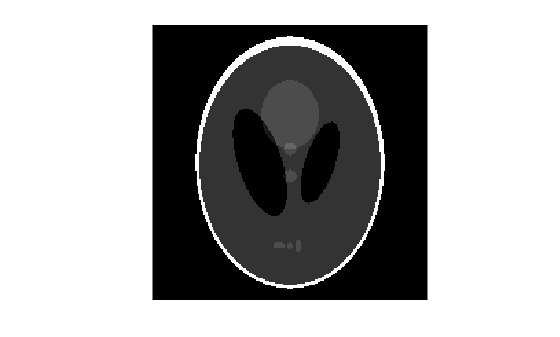# phantom

Create head phantom image

## Syntax

``P = phantom(def,n)``
``P = phantom(E,n)``
``[P,E] = phantom(___)``

## Description

example

````P = phantom(def,n)` generates an image of a head phantom that can be used to test the numerical accuracy of `radon` and `iradon` or other two-dimensional reconstruction algorithms. `P` is a grayscale image that consists of one large ellipse (representing the brain) containing several smaller ellipses (representing features in the brain). `def` specifies the type of head phantom to generate, and `n` specifies the number of rows and columns in the phantom image.```
````P = phantom(E,n)` generates a user-defined phantom, where each row of the matrix `E` specifies an ellipse in the image. `E` has six columns, with each column containing a different parameter for the ellipses.```
````[P,E] = phantom(___)` returns the matrix `E` used to generate the phantom.```

## Examples

collapse all

Create the modified Shepp-Logan head phantom image and display it.

```P = phantom('Modified Shepp-Logan',200); imshow(P)```## Input Arguments

collapse all

Type of head phantom to generate, specified as one of the following.

• `'Shepp-Logan'` — Test image used widely by researchers in tomography

• `'Modified Shepp-Logan'` — Variant of the Shepp-Logan phantom in which the contrast is improved for better visual perception

Data Types: `char` | `string`

Number of rows and columns in the phantom image, specified as a positive integer.

Data Types: `double`

Ellipses that define the phantom, specified as an e-by-6 numeric matrix defining e ellipses. The six columns of `E` are the ellipse parameters.

Column

Parameter

Meaning

Column 1

`A`

Additive intensity value of the ellipse

Column 2

`a`

Length of the horizontal semiaxis of the ellipse

Column 3

`b`

Length of the vertical semiaxis of the ellipse

Column 4

`x0`

x-coordinate of the center of the ellipse

Column 5

`y0`

y-coordinate of the center of the ellipse

Column 6

`phi`

Angle (in degrees) between the horizontal semiaxis of the ellipse and the x-axis of the image

The domains for the x- and y-axes are [-1,1]. Columns 2 through 5 must be specified in terms of this range.

Data Types: `double`

## Output Arguments

collapse all

Phantom image, returned as an `n`-by-`n` numeric matrix.

Data Types: `double`

## Tips

For any given pixel in the output image, the pixel's value is equal to the sum of the additive intensity values of all ellipses that the pixel is a part of. If a pixel is not part of any ellipse, its value is 0.

The additive intensity value `A` for an ellipse can be positive or negative; if it is negative, the ellipse will be darker than the surrounding pixels. Note that, depending on the values of `A`, some pixels can have values outside the range [0, 1].

 Jain, Anil K., Fundamentals of Digital Image Processing, Englewood Cliffs, NJ, Prentice Hall, 1989, p. 439.